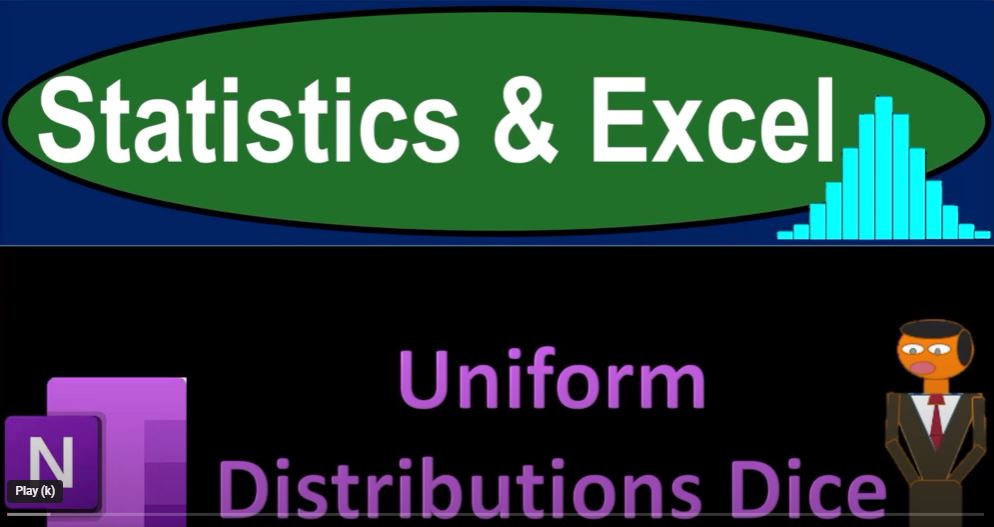## Uniform Distributions Dice 1511 Statistics & Excel

In this blog post, we’ll delve into the world of statistics and Excel, focusing on the concept of uniform distribution. While we’ll be talking about OneNote, we won’t require you to use it. So, let’s jump right into the fascinating world of data representation and predictive modeling.

Understanding Data Representation: In previous presentations, we’ve explored how to represent and describe datasets mathematically and visually, using various statistical measures like mean, median, quartiles, and graphical tools such as histograms and box-and-whisker plots. These techniques help us gain insights into data distribution and characteristics. But what if we could represent data with formulas or curves, providing us with predictive power over the dataset? That’s the goal we aim to achieve.

Introducing Uniform Distribution: To start our journey, we’ll look at the first family of curves – Uniform Distributions. Don’t be misled by the name; we’re not distributing uniforms. Instead, we’re talking about using uniform distributions as a way to represent data. For this demonstration, we’ll use dice rolls to understand how this distribution works.

Imagine you have a six-sided die, and you roll it 1000 times. What’s the likelihood of rolling any specific number (1 to 6) in a single roll? It’s 1/6, or approximately 16.67%. So, if you rolled the die 1000 times, you’d expect each number to come up around 166.67 times.

Creating a Visual Model: Keep in mind that this visualized outcome is an idealized model. In reality, you can’t roll a die 0.67 times, so this model won’t be perfect. However, it gives us predictive power over the data. When plotted, this expected outcome would form a straight line, representing a uniform distribution. The uniform distribution is essentially a family of curves, with each curve being a straight line.

Predictive Power: Even if the actual data doesn’t match the model perfectly due to randomness, having a simple formula like a straight line helps make predictions about future outcomes. Excel is a handy tool to approximate the outcomes of dice rolls. You can use the random number generator function (e.g., `=RANDbetween(1,6)`) to simulate 1000 dice rolls and compare the actual results with the expected ones.

Excel in Action: You can use Excel to count the occurrences of each number and create histograms or bar charts to visualize the data. The difference between the expected and actual results showcases the impact of randomness on the real-world data.

Running Multiple Experiments: To further illustrate the concept, you can run the dice roll experiment multiple times. Each set of results will differ slightly due to randomness, but they will all approximate a straight line. This behavior supports the idea that a uniform distribution can be represented by a simple formula.

The Big Picture: Uniform distributions are the simplest family of curves to work with, as they result in straight lines. In future presentations, we’ll explore more complex curves and formulas to represent data, giving us even more predictive power over diverse datasets.

Conclusion: Uniform distribution, despite its simplicity, demonstrates the power of using mathematical models to represent real-world data. With Excel and statistics, you can gain valuable insights and make predictions based on these models. So, stay tuned for more exciting discussions about data representation and predictive modeling in upcoming presentations!